### Excel COUNT Function Examples – Excel & Google Sheets

This Tutorial demonstrates how to use the Excel COUNT Function in Excel.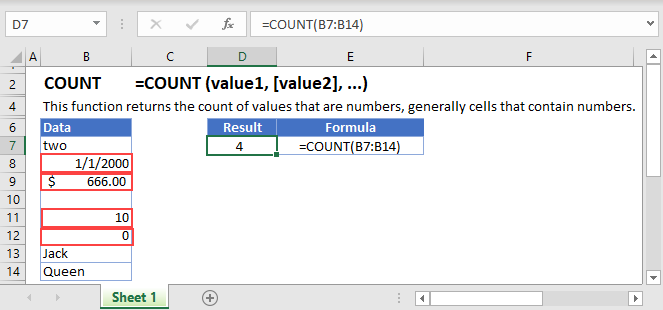# COUNT Function Overview

The COUNT function returns the count of values that are numbers, generally cells that contain numbers.

To use the COUNT Excel Worksheet Function, select a cell and type: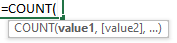(Notice how the formula inputs appear)

## COUNT function Syntax and inputs:

`=COUNT (value1, [value2], ...)`

value1 – An item, cell reference, or range.

value2 – [optional] An item, cell reference, or range

# What is the COUNT Function?

The Excel COUNT Function returns the number of cells within a given range that contain numerical values.

## How to Use the COUNT Function

To use the Excel COUNT Function, type the following:

`=COUNT(B3:B11)`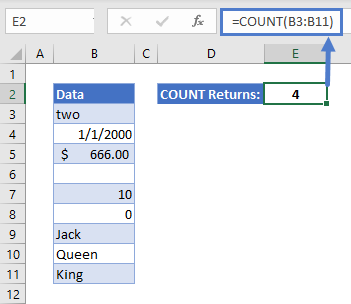In this case, COUNT returns 4, since there are four numerical values in the range B3:B11.

A few notes on this example:

• COUNT will count cells formatted as currency
• Blank cells are ignored
• Cells containing 0 are counted
AutoMacro - VBA Code Generator

## Counting Cells that Don’t Contain Numerical Data

If you need to count cells that DON’T contain numbers, you can use the formula below:

`=SUMPRODUCT(--NOT(ISNUMBER(B3:B11)))`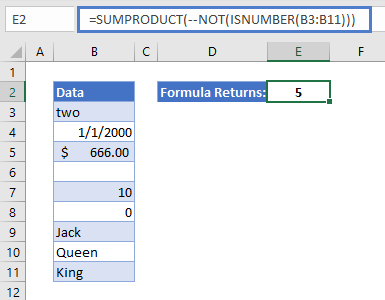Don’t worry if this formula looks a bit intimidating! We’ll work through it step-by-step:

• SUMPRODUCT multiplies two ranges together, and then adds up all the results. Here we’re just giving it one range, B3:B11. In this case it will multiply the numbers in the range by themselves (squaring them), and add up the results.
• ISNUMBER returns TRUE if the cell contains a number, and FALSE if it doesn’t.
• NOT reverses any TRUE or FALSE value that you give it.
• The double negative in front of NOT changes TRUE to 1, and FALSE to 0.
• So, take B3 for example:
• B3 contains “two”
• ISNUMBER therefore returns FALSE
• NOT flips this to TRUE
• The double negative changes this to 1
• Going through each cell in turn, what SUMPRODUCT eventually gets will be: 1,0,0,1,0,0,1,1,1
• Square all these numbers, and add up add the results, and you get 5.

## An Alternative Method

Another formula to count all cells in a range that don’t contain numerical data, is the following:

`=(ROWS(B3:B11)*COLUMNS(B3:B11)) - COUNT(B3:B11)`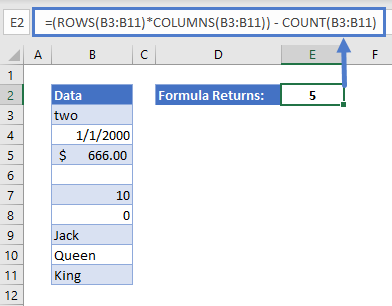In this formula:

• ROWS returns the number of rows within a given range
• COLUMNS returns the number of columns within a range
• Multiplying ROWS and COLUMNS gives us the total number of cells in the range
• Then we simply use COUNT to subtract the number of cells that DO contain numbers from the total
• This leaves us with 5 cells that don’t contain numerical data

## COUNT in Google Sheets

The COUNT Function works exactly the same in Google Sheets as in Excel: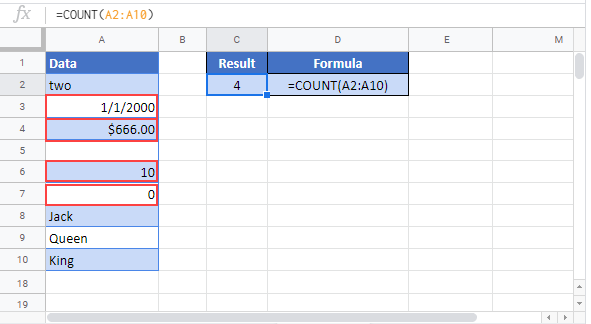## Excel Practice Worksheet

Practice Excel functions and formulas with our 100% free practice worksheets!

• Automatically Graded Exercises
• Learn Excel, Inside Excel!